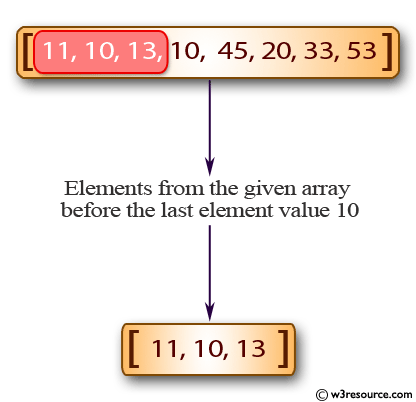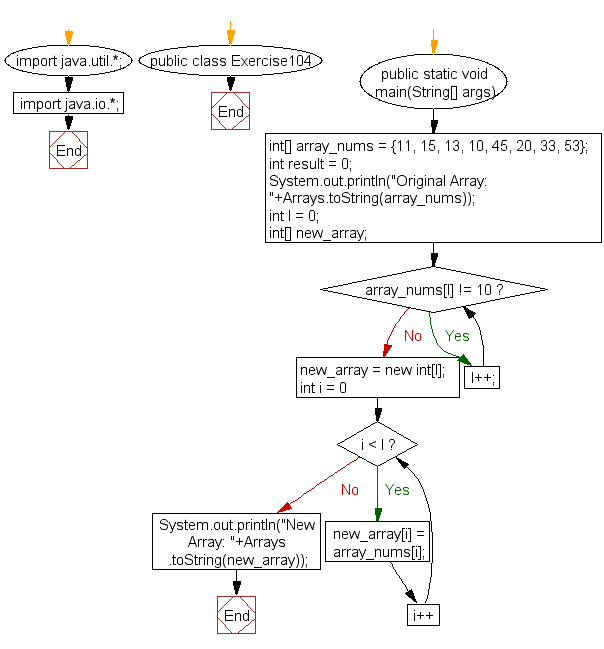﻿ Java: The array contains elements from an array before 10# Java Exercises: Create a new array from a given array of integers, new array will contain the elements from the given array before the last element value 10

## Java Basic: Exercise-104 with Solution

Write a Java program to create a new array from a given array of integers, new array will contain the elements from the given array before the last element value 10.

Pictorial Presentation:Sample Solution:

Java Code:

``````import java.util.*;
import java.io.*;
public class Exercise104 {
public static void main(String[] args)
{
int[] array_nums = {11, 15, 13, 10, 45, 20, 33, 53};
int result = 0;
System.out.println("Original Array: "+Arrays.toString(array_nums));

int l = 0;
int[] new_array;
while(array_nums[l] != 10)
l++;
new_array = new int[l];
for(int i = 0; i < l; i++)
new_array[i] = array_nums[i];
System.out.println("New Array: "+Arrays.toString(new_array));
}
}
```
```

Sample Output:

```Original Array: [11, 15, 13, 10, 45, 20, 33, 53]
New Array: [11, 15, 13]
```

Flowchart:Java Code Editor:

What is the difficulty level of this exercise?

Test your Programming skills with w3resource's quiz.

﻿

## Java: Tips of the Day

getEnumMap

Converts to enum to Map where key is the name and value is Enum itself.

```public static <E extends Enum<E>> Map<String, E> getEnumMap(final Class<E> enumClass) {
return Arrays.stream(enumClass.getEnumConstants())
.collect(Collectors.toMap(Enum::name, Function.identity()));
}
```

Ref: https://bit.ly/3xXcFZt